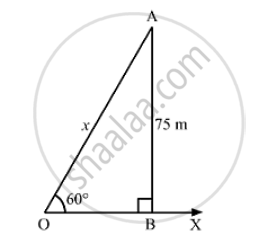# A kite is flying at a height of 75 in from the level ground, attached to a string inclined at 60°. to the horizontal. Find the length of the string, assuming that there is no slack in it. - Mathematics

A kite is flying at a height of 75 in from the level ground, attached to a string inclined at 60°. to the horizontal. Find the length of the string, assuming that there is no slack in it.
[Take sqrt(3) =1.732 ]

#### Solution

Let OX be the horizontal ground and A be the position of the kite.
Also, let O be the position of the observer and OA be the thread.
Now, draw AB ⊥ OX.
We have:
∠BOA= 60°,OA= 75m and ∠OBA = 90°
Height of the kite from the ground = AB = 75 m
Length of the string OA =  xmIn the right ΔOBA,we have:
(AB)/(OA ) = sin 60° = sqrt(3)/2
⇒ 75/x = sqrt(3)/2

⇒  x = (75xx2)/sqrt(3) = 150/1.732 = 86.6m
Hence, the length of the string is 86.6m

Concept: Heights and Distances
Is there an error in this question or solution?

#### APPEARS IN

RS Aggarwal Secondary School Class 10 Maths
Chapter 14 Height and Distance
Exercises | Q 2

Share